# 参数估计之区间估计

• A+

## 置信区间：

1. 设法构造一个样本和θ的函数G，使得其分布不依赖于未知参数，称其为枢轴量。
2. 适当的选取两个常数c,d，使得对给定的α有P（c≤G≤d）=1-α。（离散场合改为≥α）
3. 假如能将c≤G≤d进行不等式等价变形成为θ1≤θ≤θ2，则[θ1，θ2]是θ的1-α置信区间。

## 一、单个正态总体参数的区间估计

### 1.σ未知时μ的置信区间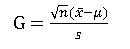1-α置信区间：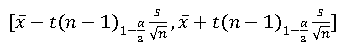1. dist = RandomReal[NormalDistribution[2, 3], 100];
2. (*采用均值为2，方差为9的正态分布样本*)
3. Needs["HypothesisTesting`"]
4. (*使用估计的函数必须加入的语句，在此声明，此为导入函数包，下文代码中此句将不再重复出现*)
5. MeanCI[dist, ConfidenceLevel -> .90]
6. (*取置信水平为90%*)
7. >>{1.2249, 2.32561}

### 2.σ已知时μ的置信区间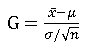1-α置信区间：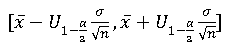mathenatica实现：

1. (*采用上个例子的样本*)
2. MeanCI[dist, KnownVariance -> 9, ConfidenceLevel -> .90]
3. >>{1.2818, 2.26871}

### 3.σ^2的置信区间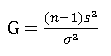1-α置信区间：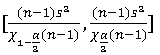mathematica实现：

1. (*采用上个例子的样本*)
2. VarianceCI[dist, ConfidenceLevel -> .90]
3. >>{5.84527, 9.34872}

## 二、双正态总体参数的区间估计

### 1.σ1^2和 σ2^2均已知时，μ1-μ2的区间估计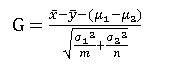1-α置信区间：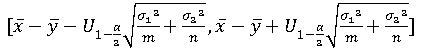mathematica实现：

1. dist1 = RandomReal[NormalDistribution[2, 3], 100];
2. dist2 = RandomReal[NormalDistribution[5, 5], 100];
3. (*产生两组不同的正态分布数据*)
4. MeanDifferenceCI[dist1, dist2, KnownVariance -> {9, 25},ConfidenceLevel -> .90]
5. (*给定两组数据的方差为9和25*)
6. >>{-3.94347, -2.02526}

### 2.σ1^2和 σ2^2未知但相等时，μ1-μ2的区间估计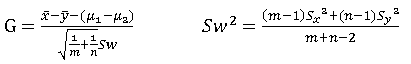1-α置信区间：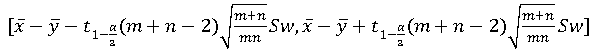mathematica实现：

1. (*采用上个例子的样本*)
2. MeanDifferenceCI[dist1, dist2, EqualVariances -> True,ConfidenceLevel -> .90]
3. >>{-3.88088, -2.08785}

### 3.σ1^2/ σ2^2的区间估计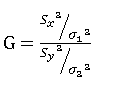1-α置信区间：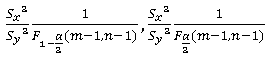mathematica实现：

1. (*采用上个例子的样本*)
2. VarianceRatioCI[dist1, dist2, ConfidenceLevel -> .90]
3. >>{0.271585, 0.527801}

## 三、其他可行函数

### 1.NormalCI

1. (*数据仍采用“一”中的*)
2. dist = RandomReal[NormalDistribution[2, 3], 100];
3. std = 3/Sqrt; (*均值的标准差*)
4. NormalCI[Mean[dist],std,ConfidenceLevel -> .90]
5. >>{1.2818, 2.26871}

### 2.StudentTCI

1. (*数据仍采用“一”中的*)
2. sstd = StandardDeviation[dist]/Sqrt; (*样本标准差*)
3. dist = RandomReal[NormalDistribution[2, 3], 100];
4. StudentTCI[Mean[dist], sstd, 100 - 1, ConfidenceLevel -> .90]
5. {1.2249, 2.32561}

### 3.ChiSquareCI

1. (*数据仍采用“一”中的*)
2. dist = RandomReal[NormalDistribution[2, 3], 100];
3. stdn = Variance[dist]; (*样本方差*)
4. ChiSquareCI[stdn, 100 - 1, ConfidenceLevel -> .90]
5. >>{8.82679, 14.1173}

### 4.FRatioCI

1. (*数据仍采用“二”中的*)
2. dist1 = RandomReal[NormalDistribution[2, 3], 100];
3. dist2 = RandomReal[NormalDistribution[5, 5], 100];
4. FRatioCI[Variance[dist1]/Variance[dist2], 100, 100,ConfidenceLevel -> .90]
5. >>{0.272042, 0.526914}

• 微信公众号
• 关注微信公众号
•• QQ群
• 我们的QQ群号
•